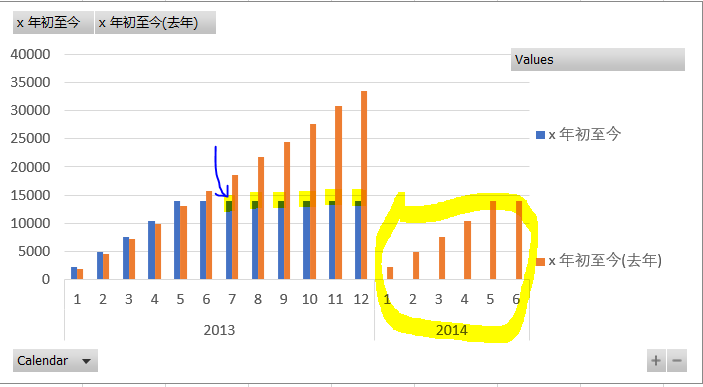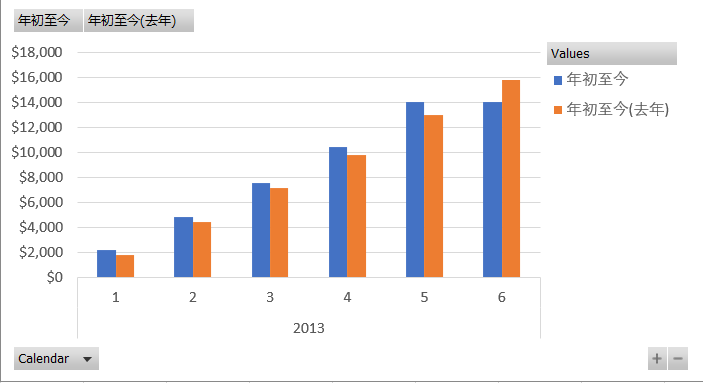o
osc_a22drz29

# DAX/PowerBI系列 - 关于时间系列 - 时间相关数值比较 - 用非自带函数

文末有彩蛋，解决蛋疼问题

• 计算年底销售总额
• 计算月度流量总额
• 进一步计算同比，环比

## 最终PowerBI效果显示如下：（耐心等待PowerBI 出来，噔噔噔噔~~~）DAX/PowerBI系列 - 参数表(Parameter Table) 度量值模板（Period Table）

## 数据模型：

Date（日期表）

Product（产品表）

Sales （销售表）

Inventory（库存表）## 要点：

``````有些盆友觉得定义这个度量值是多余的，但是细看下面你就会发现这样定义是十分有用的：
1.	易懂
2.	简化引用这个度量值的其他度量值
3.	易于维护``````

``[Amount] := SUM(Sales[SalesAmount])``

``````[Amount MTD] :=
CALCULATE (
[Amount],
FILTER (
ALL ( 'Date' ),
'Date'[Year] = MAX ( 'Date'[Year] )
&& 'Date'[Month] = MAX ( 'Date'[Month] )
&& 'Date'[Date] <= MAX ( 'Date'[Date] )
)
)``````

月初至今(上月)  LMTD

``````[Amount LMTD] :=
VAR maxSalesDate =
MAX ( 'Sales 0'[skDate] )
VAR dateRng =
FILTER ( ALL ( 'Date' ), 'Date'[Date] <= maxSalesDate )
RETURN
CALCULATE ( [Amount MTD], DATEADD ( 'Date'[Date], -1, MONTH ), dateRng )``````

dateRng: 见文末详解。

``````1 [Amount LYMTD] :=
2 VAR maxSalesDate =
3     MAX ( 'Sales 0'[skDate] )
4 VAR dateRng =
5     FILTER ( ALL ( 'Date' ), 'Date'[Date] <= maxSalesDate )
6 RETURN
7     CALCULATE ( [Amount MTD], DATEADD ( 'Date'[Date], -1, YEAR ), dateRng )``````

``````1 [MoM] :=
2 IF (
3     [Amount MTD] <> BLANK (),
4     DIVIDE ( [Amount MTD] - [Amount LMTD], [Amount LMTD] )
5 )``````

``````[LMoM] :=
IF (
[Amount MTD] <> BLANK (),
DIVIDE ( [Amount MTD] - [Amount LYMTD], [Amount LYMTD] )
)``````

``````[Sales YTD] :=
CALCULATE (
[Amount],
FILTER (
ALL ( 'Date' ),
'Date'[Year] = MAX ( 'Date'[Year] )
&& 'Date'[Date] <= MAX ( 'Date'[Date] )
)
)``````

年初至今(去年)  LYTD

``````[Amount LYTD] :=
VAR maxSalesDate =
MAX ( 'Sales 0'[skDate] )
VAR dateRng =
FILTER ( ALL ( 'Date' ), 'Date'[Date] <= maxSalesDate )
RETURN
CALCULATE ( [Amount YTD], DATEADD ( 'Date'[Date], -1, YEAR ), dateRng )``````

``````[YoY] :=
IF (
[Amount YTD] <> BLANK (),
DIVIDE ( [Amount YTD] - [Amount LYTD], [Amount LYTD] )
)``````

## ^如何在图表中显示LYTD,LMTD的数据

 没有用dateRng筛选 用dateRng筛选 ``````[x Amount YTD] := CALCULATE ( [Amount], FILTER ( ALL ( 'Date' ), 'Date'[Year] = MAX ( 'Date'[Year] ) && 'Date'[Date] <= MAX ( 'Date'[Date] ) ) )`````` ``````[Amount YTD] := VAR maxSalesDate = MAX ( 'Sales 0'[skDate] ) VAR dateRng = FILTER ( ALL ( 'Date' ), 'Date'[Date] <= maxSalesDate )RETURN CALCULATE ( [Amount], FILTER ( ALL ( 'Date' ), 'Date'[Year] = MAX ( 'Date'[Year] ) && 'Date'[Date] <= MAX ( 'Date'[Date] ) ), dateRng )``````Time-Pattern,日期度量值对比,度量值汇总, 同比,环比,YoY, MoM,库存对比,年度环比,月度环比

o

### osc_a22drz29

PowerPivot+PowerQuery+PowerBI国内文章导航

2017/04/04
0
0
【必】PowerBI 大型原创精华-5000小时50万字-饕餮盛宴 - 知乎

V 0.1.00 - 190827 - 初始发布。 前言的前言 根据与出版社编辑的交流，本文等同于一部400页的图书。本文有核心的目标，有体系化的划分，有视频教程体系的辅助，有思想的总结。 总之，这不仅仅...

PowerBI战友联盟
2019/10/21
0
0

ALLSELECTED函数 ALLSELECTED函数，被微软划分为“筛选”类函数，隶属于“表函数”。其用途，常常是用来计算或者显示明面上的筛选影响，而忽略其行上下文的影响。 如果说，CALCULATE函数是D...

0
0

TOTAL函数系列 TOTAL函数系列共包含三个函数：TOTALMTD函数，TOTALQTD函数，TOTALYTD函数。 隶属于“值函数”，用途相对于DATESMTD系列函数更为直接。 用途：用来计算月初/季度初/年初迄今的...

06/10
0
0

OPENINGBALANCEMONTH函数 OPENINGBALANCEQUARTER函数 OPENINGBALANCEYEAR函数 OPENINGBALANCE系列函数一共包含三个函数：OPENINGBALANCEMONTH函数，OPENINGBALANCEQUARTER函数，OPENINGBALA......

05/11
0
0

2019/07/23
11
0
Hacker News 简讯 2020-07-10

FalconChen

26
0
OSChina 周五乱弹 —— 求求你吃了我吧，不要再玩弄食物的感情了

Osc乱弹歌单（2020）请戳（这里） 【今日歌曲】 @巴拉迪维 ：张喆的单曲《陷阱 》 这首歌已经在网易找不到原唱了，不知道被哪家买了版权。#今日歌曲推荐# 《陷阱 》- 张喆 手机党少年们想听歌...

32
1

jackli2020

7
0
@RequestMapping, consumes 提交简单有意思的测试

getParm @GetMapping("getParm")public Result getParm(String id){ System.out.println(); return ResultFactory.success(id);} 等同于 == bodyParm @PostMapping("bodyParm......

25
0